‘You Only Look Once: Unified, Real-Time Object Detection’ (YOLO) proposed an object detection model which was presented at IEEE Conference on Computer Vision and Pattern Recognition in 2016.

# Summary

• Problem Statement
• Prior work on object detection repurposes classifiers to perform detection.
• Prior work on object detection is not fast enough or have complex multistage training pipeline.
• Research Objective
• To boost up the speed of prior object detection method while having similar performance with state-of-the-art detection systems
• Solution Proposed: YOLO
• Frame object detection as a regression problem to spatially separated bounding boxes and associated class probabilities
• A single neural network predicts bounding boxes and class probabilities directly from full images in one evaluation.
• Contribution
• YOLO is fast since the whole detection pipeline is a single network, it can be optimized end-to-end directly on detection performance.
• YOLO reasons gloablly about the image when making predictions
• YOLO learns very general representations of objects.

# YOLO

• The Process of YOLO Detection System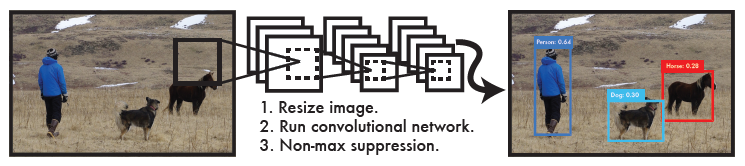1. Resizes the input image to $448 \times 448$
2. Run a single convolutional network on the image
3. Thresholds the resulting detections by the model’s confidence

## Unified Detection

YOLO uses features from the entire image to predict each bounding box and predicts all bounding boxes across all classes for an image simultaneously. This enables end-to-end training and real-time speeds while maintaining high average precision.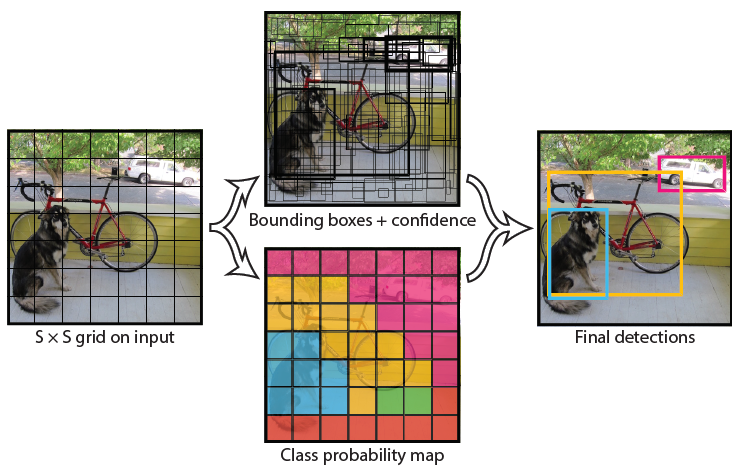• YOLO models detection as a regression problem.
• It divides the image into an $S \times S$ grid.
• For each grid cell predicts $B$ bounding boxes, confidence for those boxes, and $C$ class probabilities.
• Confidence score $Pr(Object)*IOU_{pred}^{truth}$: How confident the model is that the box contains an object and also how accurate it thinks the box is that it predicts.
• If no object exists, the confidence scores is zero. Otherwise, it is equal the Intersection Over Union (IOU) between the predicted box and the ground truth.
• Each bounding box consists of 5 predictions: $x, y, w, h, confidence$
• Each grid cell predicts $C$ conditional class probabilities, $Pr(Class_i \mid Object)$.
• At test time, we multiply the conditional class probabilities and the individual box confidence which encode both the probability of that class appearing in the box and how well the predicted box fits the object.
• These predictions are encoded as an $S \times S \times (B * 5 + C)$ tensor.

## Network Design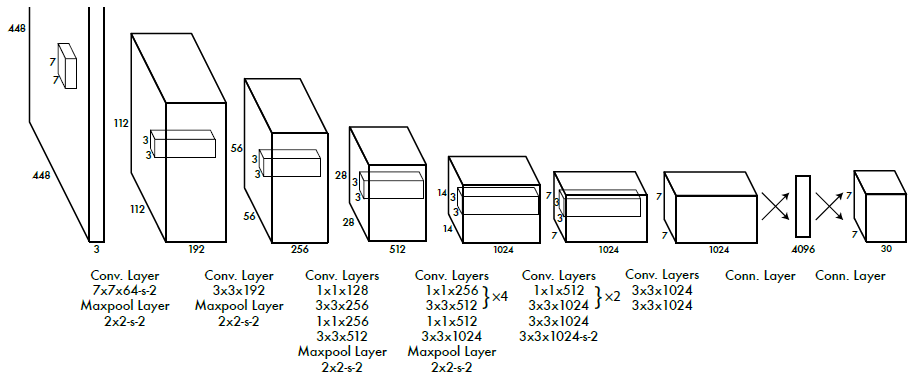• YOLO network has 24 convolutional layers followed by 2 fully connected layers.
• Alternating $1 \times 1$ convolutional layers reduce the features space from preceding layers.
• We pretrain the convolutional layers on the ImageNet at half the resolution and then double the resolution for detection.

## Training

• Final layer predicts both class probabilities and bounding box coordinates.
• YOLO use a linear activation function for the final layer and other layers use the leaky rectified linear activation.
• We optimize for sum-squared error in the output of the model since it is easy to optimize.

• Multi-part loss function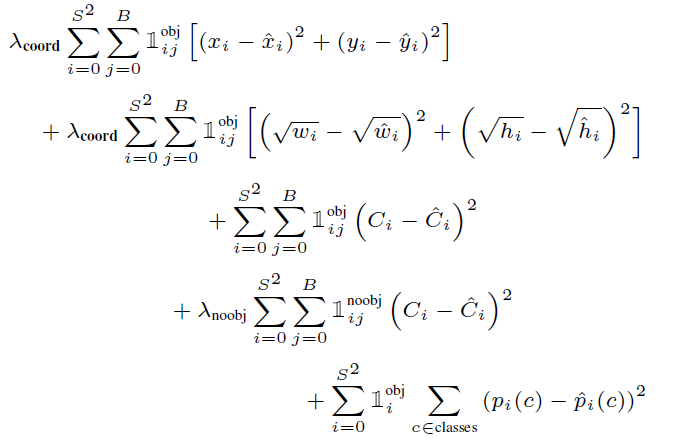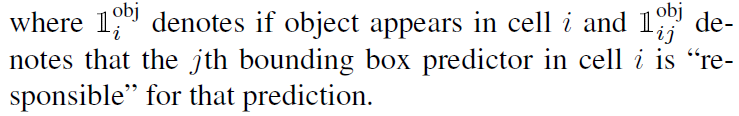• $\lambda_{coord}$: parameter for bounding box coordinate prediction
• $\lambda_{noobj}$: parameter for confidence prediction when boxes do not contain objects

# Limitations of YOLO

• YOLO imposes strong spatial constraints on bounding box predictions since each grid cell only predicts two boxes and can only have one class.
• This spatial constraint limits the number of nearby objects and small objects that appear in groups, such as flocks of birds.
• Since YOLO learns to predict bounding boxes from data, it struggles to generalize to objects in new or unusual aspect ratios or configurations.
• A small error in a large box is generally benign but a small error in a small box has a much greater effect on IOU.
• Since the loss function treats errors the same in small bounding boxes versus large bounding boxes.

# Comparison to R-CNN Series

• While Fast and Faster R-CNN offer speed and accuracy improvements over R-CNN, both still fall short of real-time performance.
• Instead of trying to optimize individual components of a large detection pipeline, YOLO throws out the pipeline entirely and is fast by design.
• YOLO is a general purpose detector that learns to detect a variety of objects simultaneously.

# Experiment

## Real-Time Systems on PASCAL VOC 2007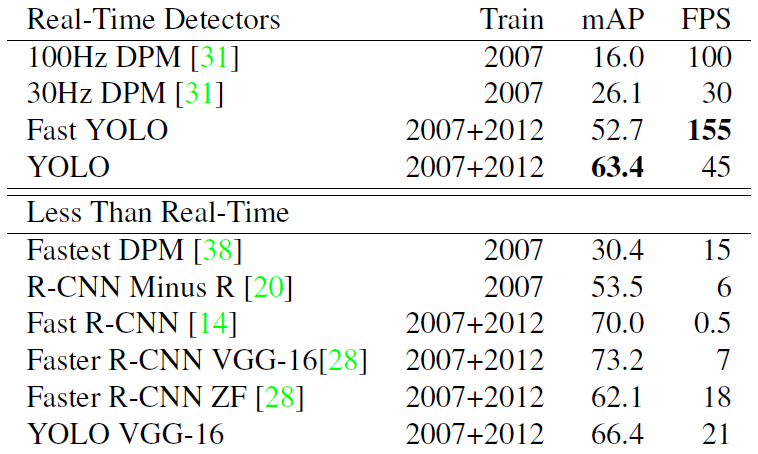• Fast YOLO is the fastest detedtor on record and is still twice as accurate as any other real-time detector.
• YOLO is 10 mAP more accurate than the fast version while still well above real-time in speed.

## Error Analysis: Fast R-CNN vs. YOLO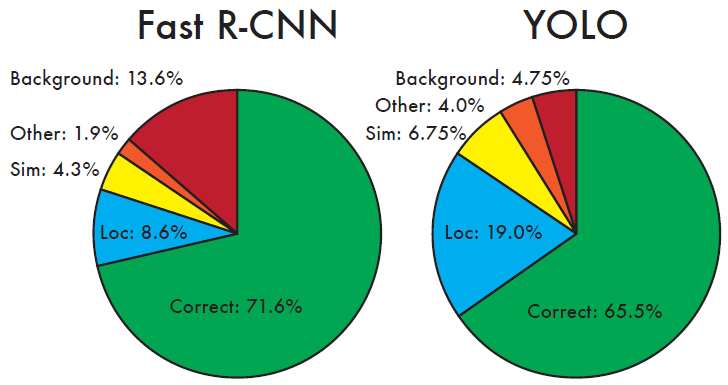• Show the percentage of localization and background errors in the top N detections for various categories.
• YOLO struggles to localize objects correctly.
• Fast R-CNN makes much fewer localization errors but far more background errors.

## Model combination experiments on VOC 2007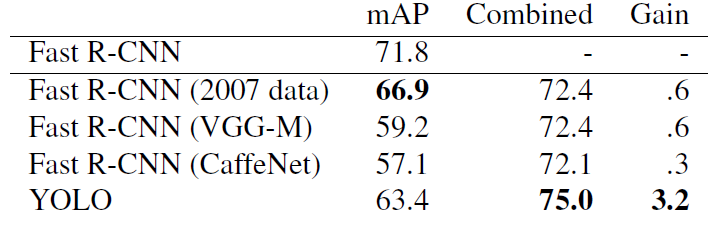• Other vesions of Fast R-CNN provide only a small benefit while YOLO provides a significant performance boost.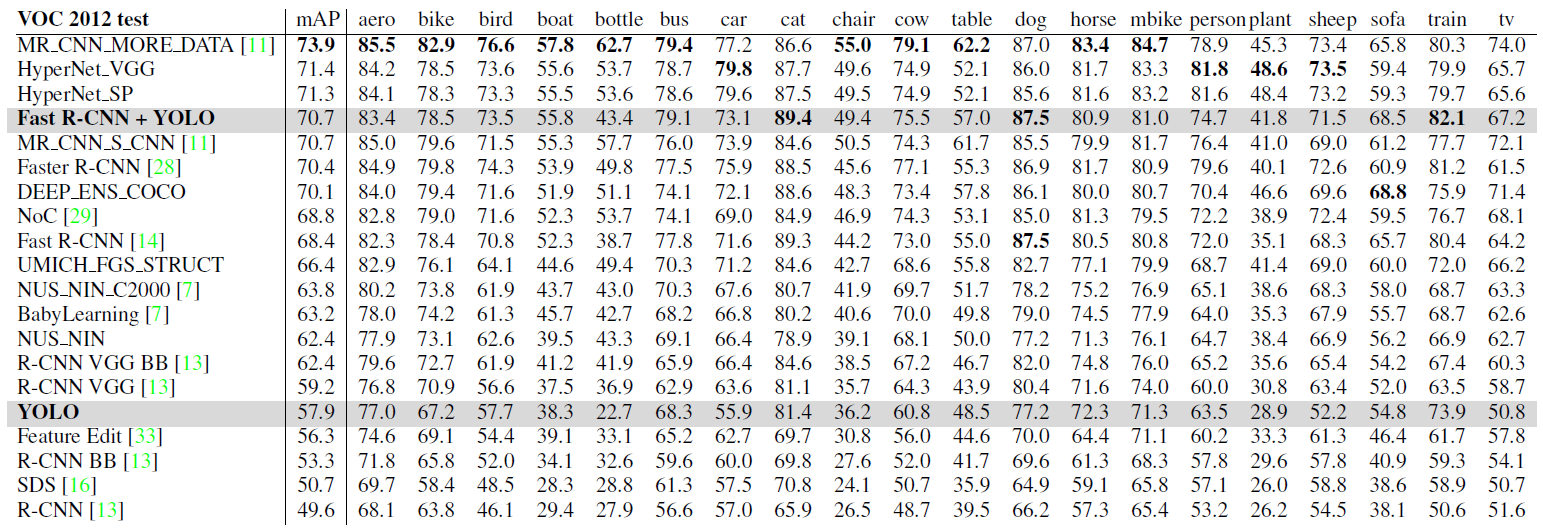• YOLO is the only real-time detector and Fast R-CNN + YOLO is the forth highest scoring method.

## Generalization results on Picasso and People-Art datasets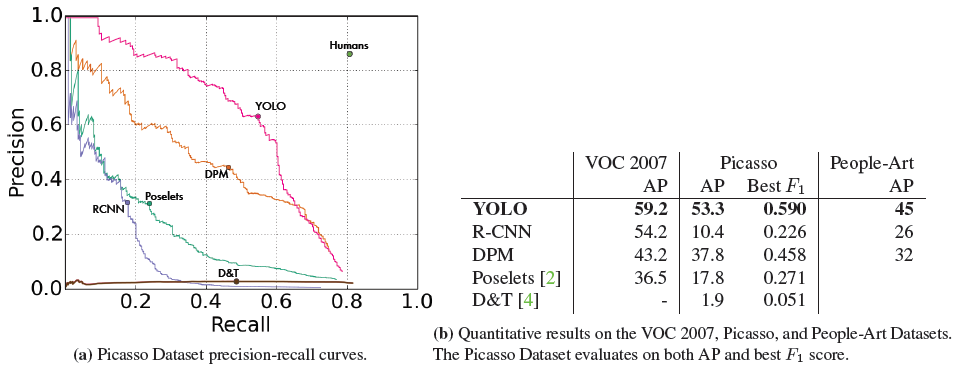• YOLO has good performance on VOC 2007 and its AP degrades less than other methods when applied to artwork.

## Qualitative Results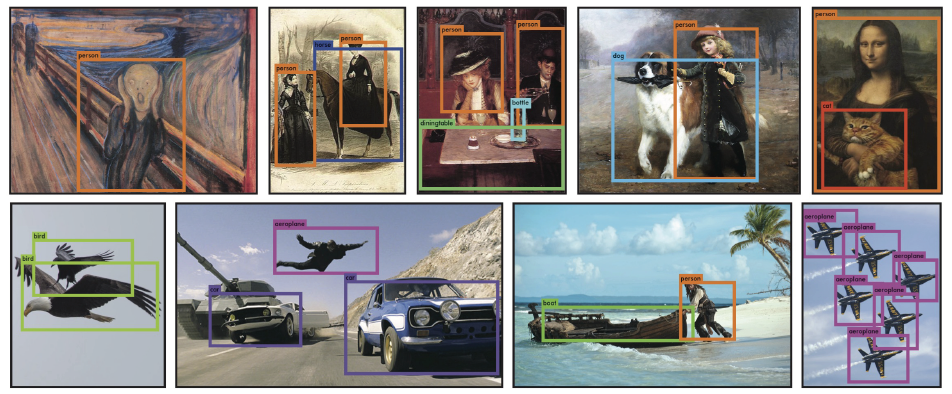• YOLO running on sample art work and natural images from the internet and it is mostly accurate although it does think one person is an airplane.
• Paper: You Only Look Once: Unified, Real-Time Object Detection [Link]